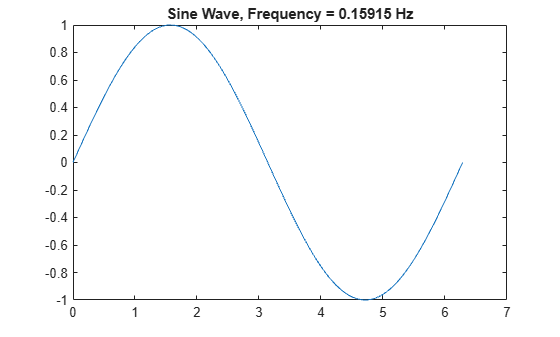# Convert Numeric Values to Text

This example shows how to convert numeric values to text and append them to larger pieces of text. For example, you might want to add a label or title to a plot, where the label includes a number that describes a characteristic of the plot.

### Convert to Strings

Before R2016b, convert to character vectors using `num2str`.

To convert a number to a string that represents it, use the `string` function.

`str = string(pi)`
```str = "3.1416" ```

The `string` function converts a numeric array to a string array having the same size.

```A = [256 pi 8.9e-3]; str = string(A)```
```str = 1x3 string "256" "3.141593" "0.0089" ```

You can specify the format of the output text using the `compose` function, which accepts format specifiers for precision, field width, and exponential notation.

`str = compose("%9.7f",pi)`
```str = "3.1415927" ```

If the input is a numeric array, then `compose` returns a string array. Return a string array that represents numbers using exponential notation.

```A = [256 pi 8.9e-3]; str = compose("%5.2e",A)```
```str = 1x3 string "2.56e+02" "3.14e+00" "8.90e-03" ```

Before R2016b, convert numbers to character vectors and concatenate characters in brackets, `[]`.

The simplest way to combine text and numbers is to use the `plus` operator (`+`). This operator automatically converts numeric values to strings when the other operands are strings.

For example, plot a sine wave. Calculate the frequency of the wave and add a string representing that value in the title of the plot.

```X = linspace(0,2*pi); Y = sin(X); plot(X,Y) freq = 1/(2*pi); str = "Sine Wave, Frequency = " + freq + " Hz"```
```str = "Sine Wave, Frequency = 0.15915 Hz" ```
`title(str)`Sometimes existing text is stored in character vectors or cell arrays of character vectors. However, the `plus` operator also automatically converts those types of data to strings when another operand is a string. To combine numeric values with those types of data, first convert the numeric values to strings, and then use `plus` to combine the text.

`str = 'Sine Wave, Frequency = ' + string(freq) + {' Hz'}`
```str = "Sine Wave, Frequency = 0.15915 Hz" ```

### Character Codes

If your data contains integers that represent Unicode® values, use the `char` function to convert the values to the corresponding characters. The output is a character vector or array.

```u = [77 65 84 76 65 66]; c = char(u)```
```c = 'MATLAB' ```

Converting Unicode values also allows you to include special characters in text. For instance, the Unicode value for the degree symbol is 176. To add `char(176)` to a string, use `plus`.

```deg = char(176); temp = 21; str = "Temperature: " + temp + deg + "C"```
```str = "Temperature: 21°C" ```

Before R2016b, use `num2str` to convert the numeric value to a character vector, and then concatenate.

`str = ['Temperature: ' num2str(temp) deg 'C']`
```str = 'Temperature: 21°C' ```

Since R2019b

You can represent hexadecimal and binary values in your code either using text or using literals. The recommended way to represent them is to write them as literals. You can write hexadecimal and binary literals using the `0x` and `0b` prefixes respectively. However, it can sometimes be useful to represent such values as text, using the `dec2hex` or `dec2bin` functions.

For example, set a bit in a binary value. If you specify the binary value using a literal, then it is stored as an integer. After setting one of the bits, display the new binary value as text using the `dec2bin` function.

`register = 0b10010110`
```register = uint8 150 ```
`register = bitset(register,5,0)`
```register = uint8 134 ```
`binStr = dec2bin(register)`
```binStr = '10000110' ```